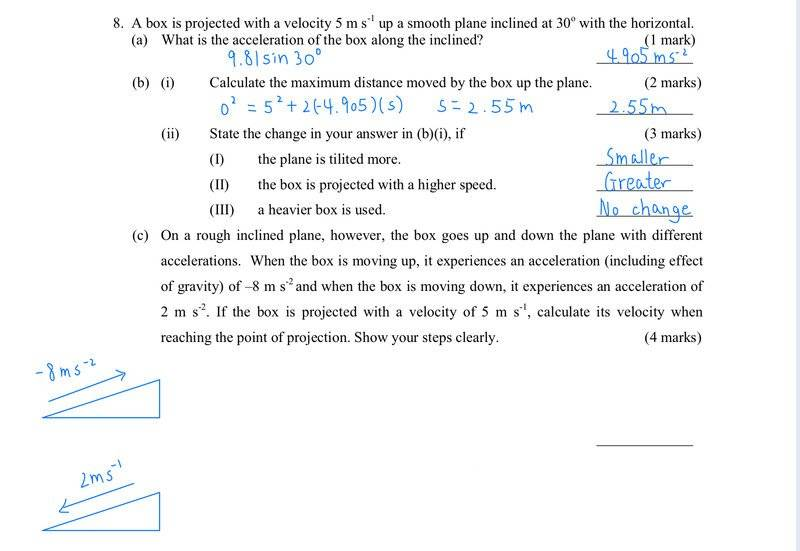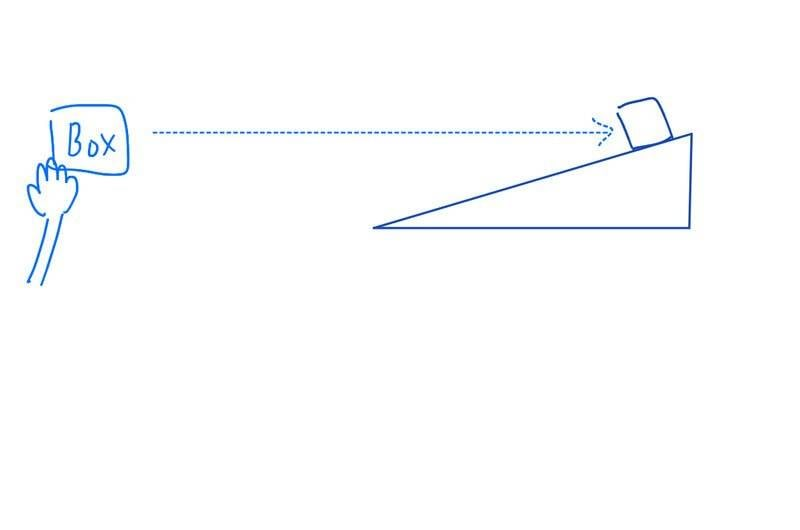# SUVAT Problem: Box moving on inclined plane

• dorothy
In summary, the conversation is discussing a physics problem involving a box being projected horizontally and landing on an inclined plane. The conversation addresses the question of how far up the plane the box goes and its velocity at that point. The participants also discuss the correct units and conventions for acceleration and velocity in this situation.f

#### dorothy

Homework Statement
Does anyone knows how to solve these questions? Thanks a lot!
Relevant Equations
v=u+at
s=ut+1/2at^2
v^2=u^2+2asLast edited:
You seem to already have solved everything except (c), for which you have not shown any effort. What are your own thoughts regarding (c)?

Homework Statement:: Does anyone knows how to solve these questions? Thanks a lot!
Relevant Equations:: v=u+at
s=ut+1/2at^2
v^2=u^2+2as

View attachment 299486
In part c, how far up the plane does the box go? What is its velocity at this point?

You seem to already have solved everything except (c), for which you have not shown any effort. What are your own thoughts regarding (c)?
Hi, I want to whether my answers on a & b are correct or not? For (c), I don’t really get the question, like what is the point of projection? How’s the deceleration and acceleration do in this case (c)? Thank you.

In part c, how far up the plane does the box go? What is its velocity at this point?
Before that, I want to ask whether I understand question8 correctly? Is it true that there is a guy throwing(projecting) a box horizontally and the box finally land on the inclined plane(like what I have drawn)? Thank you.Hi, I want to whether my answers on a & b are correct or not?
I don't agree with your answer to a). Acceleration is a vector, not a scalar.

This also affects the answer I would give to b) i) I).

Thaty said, it's not clear that the question setter acknowledges that acceleration is a vector.

•dorothy
I don't agree with your answer to a). Acceleration is a vector, not a scalar.

This also affects the answer I would give to b) i) I).

Thaty said, it's not clear that the question setter acknowledges that acceleration is a vector.
Should (a) be -4.905?

Should (a) be -4.905?
That's what I would put. With the units as you have in your answer.

•dorothy
PS It does say velocity ##5 \ m/s## up the incline, which infers a convention that up the incline is positive. I'd prefer, however, that the question setter be more explicit about this. E.g. say a velocity of ##+5 \ m/s## up the incline. Then there is no doubt.

That's what I would put. With the units as you have in your answer.
I see. What about (b)? Are all of them correct?

I see. What about (b)? Are all of them correct?
I don't agree with b i) I).

Before that, I want to ask whether I understand question8 correctly? Is it true that there is a guy throwing(projecting) a box horizontally and the box finally land on the inclined plane(like what I have drawn)? Thank you.
View attachment 299489
I expect the exercise composer means ##5## m/s along the incline, not ##5## m/s in a horizontal diertion.##\ ##

•Chestermiller and PeroK
If we look at part c) it's clear that up is positive. Note, however, that the question setter gets confused with motion down the slope and changes the convention. The acceleration down the slope should be ##-2 \ ms^{-2}##.

This seems to be a common cause of confusion: that negative acceleration is reducing speed (deceleration) and positive acceleration is increasing speed. But, if you think about it, this is not correct. Once we have established that up is positive, then the acceleration of gravity is ##-9.8 \ ms^{-2}## throughout projectile motion. It doesn't change to ##+9.8 \ ms^{-2}## for the descent. And, in fact, the SUVAT equation would no longer work if you change your convention half way through.

In this case, the acceleration does change so you have to reset your SUVAT equations. But, I suggest there is no need to change convention and make down positive.

I don't agree with b i) I).

May I know why bi) is not correct? I don’t know how to do it.

May I know why bi) is not correct? I don’t know how to do it.
Sorry I meant b ii) I.

The acceleration has a greater magnitude in this case.

Sorry I meant b ii) I.

The acceleration has a greater magnitude in this case.
So higher acceleration gives smaller distance traveled until it turns around ... as OP stated.

Sorry I meant b ii) I.

The acceleration has a greater magnitude in this case.
But if i assume the theta is 45° (which is greater than 30°, tilited more), then i put it into -9.81sin45°. I get the new acceleration=-6.9ms^-2. Next, I put 6.9 into the equation and get the new max distance =1.8 which is smaller than the original 2.55

But if i assume the theta is 45° (which is greater than 30°, tilited more), then i put it into -9.81sin45°. I get the new acceleration=-6.9ms^-2. Next, I put 6.9 into the equation and get the new max distance =1.8 which is smaller than the original 2.55
That right. It's like having more powerful brakes: you stop in a shorter distance.

So higher acceleration gives smaller distance traveled until it turns around ... as OP stated.
So if the plane is tilited more, it becomes steeper, the distance traveled will also become smaller. Does it mean that I am actually correct on bii)1)?

That right. It's like having more powerful brakes: you stop in a shorter distance.
Does it mean that bii) I) is correct ?

Does it mean that bii) I) is correct ?

No worries :) For part c, can you explain to me what is the meaning of reaching the point of projection? I don’t know which point it is. I’m super confused now

No worries :) For part c, can you explain to me what is the meaning of reaching the point of projection? I don’t know which point it is.
It was my fault.

I can only imagine that it means back at the bottom of the incline!

No worries :) For part c, can you explain to me what is the meaning of reaching the point of projection? I don’t know which point it is. I’m super confused now
Probably the point where it was released with the original speed, but on the way down.

It was my fault.

I can only imagine that it means back at the bottom of the incline!

Thank you for your help! :)